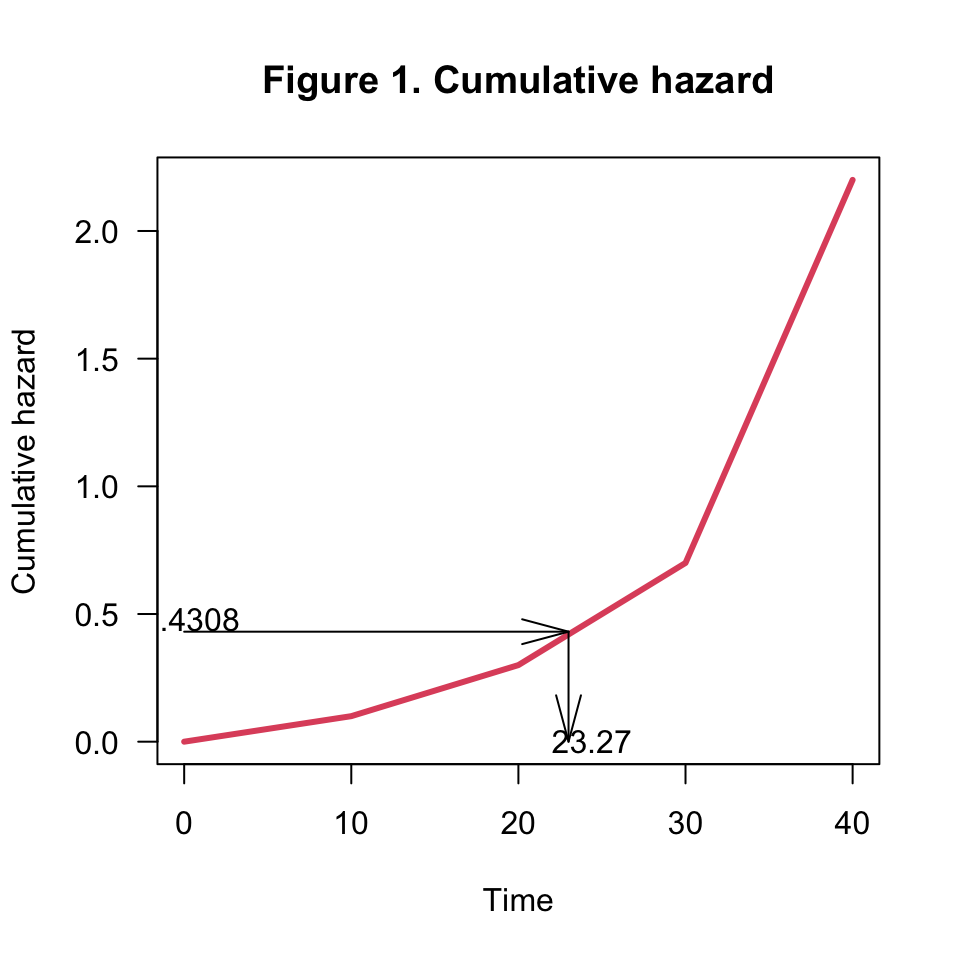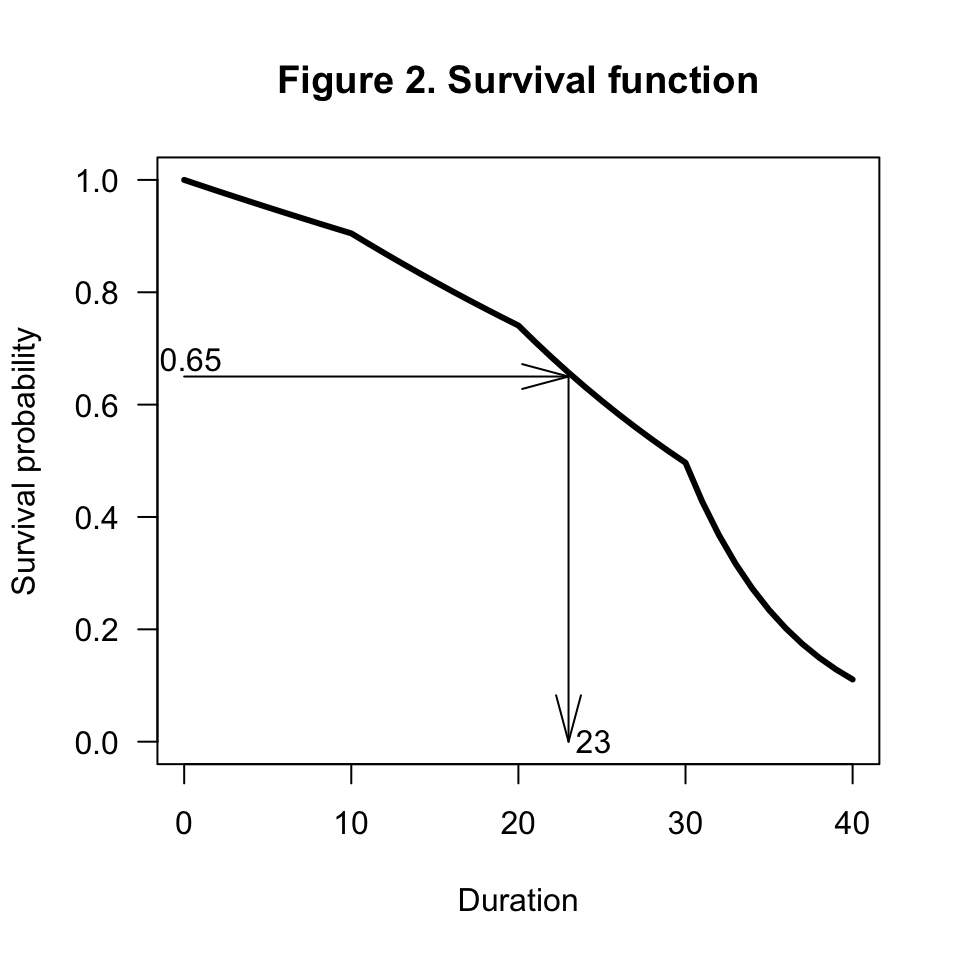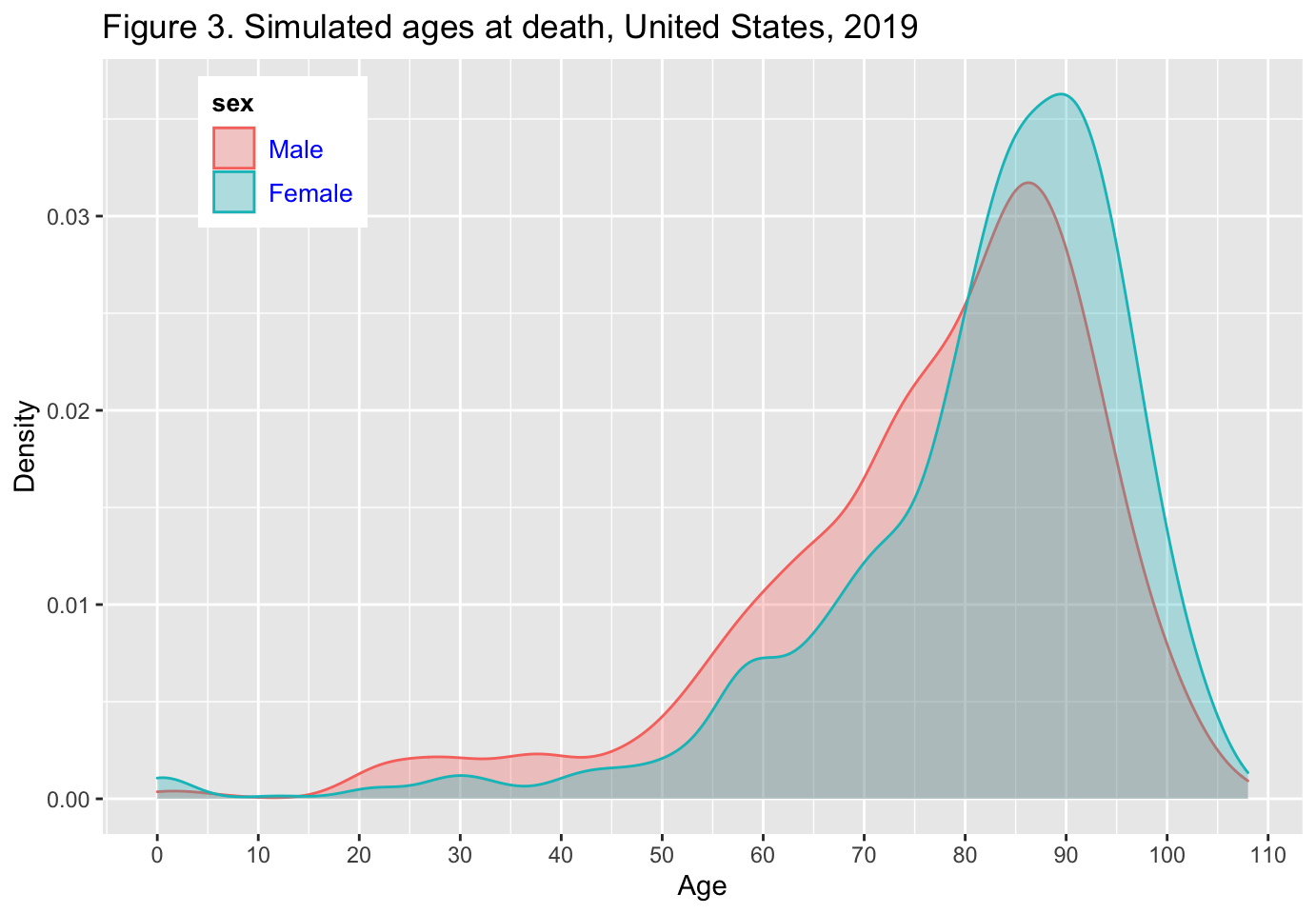## Introduction

Life histories are sequences of transitions between states of existence Willekens (2014). In stochastic simulation of life histories, ages at transition are obtained by sampling time-to-event or waiting time distributions.The time-to-event is the duration between a reference event,e.g. birth, and the event of interest. In survival analysis, it is known as the survival time. A number of parametric models of survival times have been proposed in the literature. They include the exponential distribution, the Gompertz distribution and the Weibull distribution. The piecewise-exponential distribution is an extension of the exponential distribution. The exponential waiting time distribution has a single parameter, the constant rate of transition, hazard rate or incidence rate. In the piecewise-exponential model the transition rates are piecewise-constant. They are constant during time intervals and vary between intervals. Piecewise-constant transition rates are common in demography because transition rates are often published by age or age group.

The piecewise-exponential waiting time distribution is the subject of this vignette. By sampling the distribution, individual waiting times can be generated. In the application, two states of existence are considered: alive and dead. The event of interest is death. The transition rate is the death rate.

An extension of the two-state model to a multistate model is discussed in the vignette entitled “Simulation of life histories”. The basic approach to sampling waiting time distributions remains the same, however.

The generic approach to generating waiting times consists of two steps (Rubinstein and Kroese 2017). In the first step,a random number is drawn from the standard uniform distribution U(0,1). The random draw is a value between zero and one, interpreted as a probability and denoted by u. The second step is to determine the duration at which the probability of a transition is u. The waiting time is denoted by the random variable X with realization x.

Several R functions combine the two steps. They facilitate the simulation of waiting times to transition and ages at transition. A selection of functions are listed in this vignette.

The vignette consists of two sections. The first is a brief theoretical background. The key concept is the inverse cumulative distribution function or quantile function. Concepts are illustrated using the exponential distribution and the piecewise-exponential distribution, and a few numerical illustrations should clarify the sampling of distribution. The second section is an application. Individual ages at death (lifespans) are generated from period age-specific death rates. The death rates are retrieved from the Human Mortality Database (HMD). The retrieval of data from the HMD is described in the tutorial, which is the third vignette that comes with the package $$VirtualPop$$.

## Theoretical background: simulating waiting times

### Overview

Let X be a continuous random variable denoting the waiting time to a transition (or time-to-transition) and let x be a realization of X. The cumulative distribution function (cdf) of X is ${F_X(x)=Pr\{X \le x\}=1-exp\left[-\int_0^x\mu(\tau) d\tau \right]=1-exp \left[-\Lambda(x) \right]}\tag{1}$

with $$\mu(\tau)$$ the instantaneous hazard rate at duration $$\tau$$ and $$\Lambda(x)=\int_0^x\mu(\tau) d\tau$$ the cumulative or integrated hazard. The cumulative hazard depends on $$\mu(\tau)$$ and the exposure time $$d\tau$$. The complement of the cumulative distribution function is the survival function: $$S_X(x)=exp \left[-\Lambda(x) \right]$$.

$$F_X(x)$$ is the probability that the value of X is smaller than or equal to a given value x. It is a nondecreasing function of x. The inverse cumulative distribution function $$F_X^{-1}$$ gives the value of x that makes $$F_X(x)$$ equal to a given probability, u say. The inverse cumulative distribution function is also known as the quantile function. The quantile functions is the workhorse of stochastic simulation. If the function $$F_X (x)=u$$ has no analytical solution, the value if $$x_u$$ must be determined numerically, which involves iteration.

Since $$F_X (x)$$ is a nondecreasing function of x, the inverse cumulative distribution of quantile function may be defined as

$x_u=F_X^{-1} (u)=min\{x:F_X (x) \ge u\} \tag{2}$ It is the lowest value of x for which the cumulative distribution function is equal to or exceeds u.

First, we consider a simple example. The general case is covered next. Assume the waiting time to a transition is exponentially distributed. The exponential distribution has a single parameter, the transition rate or hazard rate $$\mu$$. The rate is constant. The survival function is $$S_X(x)=exp\left [ - \mu x\right]$$ and the cumulative distribution function is $$F_X(x)=1-S_X(x)$$. The value of x at which $$F_X(x)=u$$ is

$x_u=F_X^{-1}(u)=-\frac{ln(1-u)}{\mu} \tag{3}$ A random draw from the exponential distribution with parameter $$\mu$$ is equivalent to a random draw from the standard uniform distribution, followed by the computation of x_u using equation (3). The method is the most fundamental approaches for sampling from a desired distribution. Rubinstein and Kroese (2017, 55) refer to the method as the inverse-transform method. See also Taimre, Kroese, and Botev (2019).

Now consider the general case. Recall that $$x=x_u$$ if the following condition is satisfied: $$\Lambda(x)=-ln(1-u)$$ or

$g(x)=\Lambda(x)+ln(1-u)=\int_{0}^{x}{\mu(\tau) d\tau}+ln(1-u)=0 \tag{4}$

The value of x that satisfied the condition is the root of equation $$g(x)=0$$. The root is denoted by $$x_u$$.

In R, the function $$runif(n)$$ draws n random numbers from the standard uniform distribution. The function $$rexp(n,rate)$$ of the R Base system draws n random waiting times from the exponential distribution with parameter $$rate$$ ($$rate=\mu$$). The following code draws 10 random waiting times from the exponential distribution. The code is followed by the result.

rexp(n=10,rate=0.1)
#>    0.6772223  6.0110470 11.4073575 18.3563053  5.8582105 37.3953449
#>   15.3517189  4.7740699  7.1528561 24.9989641

The generic function $$uniroot()$$ is often used to obtain the root of the equation $$g(x)=0$$.

### The piecewise-exponential distribution

A piecewise constant hazard rate is a sequence of constant hazard rates. The hazard rate is constant in an interval and varies between intervals. The cumulative hazard at the end of an interval is the hazard rate during that interval times the length of the interval. Let $$\mu(t_0,t_1 )=\mu_1$$ be the hazard rate during the interval that starts at $$t_0$$ and ends at $$t_1$$ and $$\mu(t_1,t_2 )=\mu_2$$ the hazard rate from $$t_1$$ to $$t_2$$. In general, the hazard rate during the interval $$(t_i, t_{i+1})$$ is $$\mu_{i+1}$$. The time at which the hazard rate changes is a break point. The cumulative hazard at some time x during the third interval is

$\Lambda(x)=\mu_1*(t_1-t_0 )+\mu_2*(t_2-t_1 )+\mu_3*( x-t_2)$ with $$\mu_i$$ the hazard rate during interval $$t_i$$ and $$t_i-t_{i-1}$$ the exposure during interval i. Since the end-point x is somewhere in the third interval, the exposure during that interval is part of the interval. The probability of an event before x is $$F_X (x)=1-exp[-\Lambda(x)]$$.

The duration x at which the event has occurred with probability u is the value of x, denoted $$x_u$$, at which $$F_X(x)=1-exp[-\Lambda(x)]=u$$. That condition is satisfied when the cumulative hazard $$\Lambda(x)=-ln(1-u)$$. If u is a random draw from a standard uniform distribution, then x_u is the value of x at which $$\Lambda(x)=-ln(1-u)$$.

Consider a period of total length 60 time units. The minimum duration is 0 and the maximum duration is 60. Assume the period is divided into four intervals: 0-10, 10-20, 20-30, and 30-60. The break points are 10, 20 and 30. Let the hazard rates be 0.1, 0.2, 0.4, 0.15. The function $$Rate\_pw()$$ retrieves the event rates at time points included in the vector $$t$$:

Rate_pw <- function (t,breakpoints,rates)
{ int <- findInterval(t,breakpoints,all.inside=TRUE)
z <- rates[int]
sojournInt <- t-breakpoints[int]
h <- data.frame(t=t,rate=z,interval=int,startInt=breakpoints[int],sojourn=sojournInt)
return(h)
}

Consider a single point in time, e.g. $$t=18.3$$. The hazard rate at time $$t=18.3$$ is

breakpoints <- c(0, 10, 20, 30, 60)
rates <- c(0.01,0.02,0.04,0.15)
Rate_pw(t=18.3,breakpoints,rates)

The function $$Rate\_pw()$$ returns a data frame with five columns: the values of t, the rate at t, the interval in which t is located, the starting time of the interval, and the sojourn time in the (current) interval.

If t is a vector, i.e. t =c(10,18.3,23.6,54.7), then

Rate_pw(t=c(10,18.3,23.6,54.7),breakpoints,rates)  

If the hazard rate is piecewise constant, then the cumulative hazard increases linearly in duration intervals. The function $$H\_pw()$$ of the $$VirtualPop$$ package computes the cumulative hazard at time t. The following function call produces cumulative hazards at time 10, 18.3, 23.6 and 54.7:

VirtualPop::H_pw(t=c(10,18.3,23.6,54.7),breakpoints,rates)
#>  0.100 0.266 0.444 4.405

Suppose we want to determine the duration at which the probability that the event has occurred is 35 percent ($$u=0.35$$). The cumulative hazard at that point in time is $$\Lambda(x)=-ln(1-0.35)=0.4308$$. The value of x at which the cumulative hazard is 0.4308 is $$\int_0^{x_u}\mu(\tau) d\tau=23.27$$ (Figure 1). The cumulative hazard at duration (quantile) 23.27 is H_pw(t=23.27,breakpoints,rates)=0.4308. Hence $$x_u=23.27$$. The cumulative distribution function at duration 23.27 is $$1-exp(-0.4308)=0.35$$. Figure 1 shows the cumulative hazard associated with the breakpoints and rates defined above.

t <- 0:40
H <- VirtualPop::H_pw(t=t,breakpoints,rates)
plot(t, H, type="l", lwd=3, col=2,xlab="Time",ylab="Cumulative hazard",las=1)
title(main="Figure 1. Cumulative hazard")
yy <- -log(1-0.35)
z <- which.min(abs(H-yy))
arrows (x0=c(0,t[z]),y0=c(yy,yy),x1=c(t[z],t[z]),y1=c(yy,0),angle=15,lty=1)
text(x=0.4,y=yy+0.05,labels=as.character (round(yy,4)))
text(x=t[z]+1.4,y=0,labels="23.27")Since the survival function can be derived unambiguously from the cumulative hazard function, one could (in principle) use the survival function to derive $$x_u$$. The survival function is $$S_X (x)=exp[-\Lambda(x)]$$. The survival probability that is consistent with u=0.35 is $$S_X (x_u )=1-0.35=0.65$$.

x <- t
plot(x, exp(-H), type="l",lwd=3,las=1,xlab="Duration",ylab="Survival probability",ylim=c(0,1))
title (main="Figure 2. Survival function")
yy <- 0.65
z <- which.min(abs(exp(-H)-yy))
arrows (x0=c(0,x[z]),y0=c(yy,yy),x1=c(x[z],x[z]),y1=c(yy,0),angle=15)
text(x=0.4,y=yy+0.03,labels=as.character (round(yy,2)))
text(x=x[z]+1.5,y=0,labels=x[z])Let’s now illustrate how to get the root of (4). The R code that finds the root of the function includes the following components:

• An R function with the equation $$g(x)=0$$ to be solved (root x should be determined).
• The endpoints of the interval to be searched for the root x. The endpoints define the lowest value of x (the first breakpoint) and the highest value of x (the last breakpoint) that will be considered as possible values of the root of the equation $$g(x)=0$$.
• A function call to $$uniroot()$$ to find the value of x ($$x_u$$) that solves equation $$g(x)=0$$.

The following function defines equation $$g(x)=0$$. Note that the survival function is used here, hence u is the survival probability and NOT the cumulative distribution; see equation 4):

pw_root <- function(t, breakpoints,rates, uu){
aa <- VirtualPop::H_pw(t, breakpoints,rates) + log(uu)
# Cum hazard rate should be equal to - log(u), with u a value of survival function
return(aa)
}
pw_root (t= c(10,18.3,23.6,54.7),breakpoints,rates,uu=0.43)
#>  -0.7439701 -0.5779701 -0.3999701  3.5610299

The function $$pw\_root()$$ is based on $$pw.root$$ written by Zinn for root finding in the package $$MicSim$$ (Zinn 2014). MicSim is an R package for the simulation of individual life histories in the context of population projection. It is an simplified R version of the MicCore (Zinn and Gampe 2009), developed as part of the MicMac project (Gampe et al. 2007).

Define the endpoints of the interval and call $$uniroot()$$:

interval=c(breakpoints,breakpoints[length(breakpoints)])
uniroot(f=pw_root,interval=interval,breakpoints,rates,uu=0.65)
#> $root #>  23.26957 #> #>$f.root
#>  -5.551115e-17
#>
#> $iter #>  7 #> #>$init.it
#>  NA
#>
#> $estim.prec #>  6.103516e-05 Now we have the tool to create a sample of piecewise-exponentially distributed random waiting times. It consists of two steps: (a) draw a random number, u say, from a standard uniform distribution and (b) solve equation (4). The function $$r.pw\_exp()$$ of $$VirtualPop$$ draws a sample of n random waiting times from a piecewise-exponential waiting time distribution with end points 0 and 60, breakpoints 10, 20 and 30, and hazard rates 0.01, 0.02, 0.04 and 0.15: pw_sample <- VirtualPop::r.pw_exp (n=1000, breakpoints, rates=rates) The object $$pw\_sample$$ is a vector of waiting times. Note that $$uniroot()$$ only works when the value of $$g(x)$$ at the lowest value of x (lowest breakpoint) has a different sign then the value of $$g(x)$$ at the highest value of x (highest breakpoint). If the function values at the endpoints are not of opposite signs (or zero), $$uniroot()$$ gives the error message “Error in uniroot(f = pw_root, interval = interval),: $$f()$$ values at end points not of opposite sign”, where $$f()$$ is $$g(x)$$. The error is caused by a very low survival probability u, which generates a time to transition that is beyond the interval, i.e. beyond the highest breakpoint. If such an error message occurs, the highest breakpoint should be increased. An alternative is to add $$extendInt=”yes”$$ as an argument of the $$uniroot()$$ function. In the implementation of $$uniroot()$$ in computation of waiting times, the computation does not stop when an error occurs, but the waiting time is given the value NA. To prevent to programme from crashing, the function $$r.pw_exp()$$ uses the $$tryCatch()$$ wrapper. The wrapper generates a value of 5000 if the endpoints are not of opposite signs. It allows the user to take appropriate action. An alternative approach to sampling a piecewise-constant exponential distribution is to use the function $$rpexp()$$ of the $$msm$$ package (Jackson 2011). The package includes a set of functions ($$pexp()$$) to compute the density, the cumulative distribution and the quantile function of the piecewise-exponential distribution. It also includes a function to generate random numbers. The function $$qpexp()$$ computes the duration at which the probability that the event has occurred is u and the survival probability is 1-u. The duration at which the survival probability is 65 percent is 23.27 units of time. It is computed using the code: msm::qpexp((1-0.65),rates,breakpoints[-length(breakpoints)]) #>  23.26957 To generate 100 random waiting times, use the code: pw.sample.msm <- msm::rpexp (n=100, rate=rates, t=breakpoints[-length(breakpoints)]) mean(pw.sample.msm) #>  26.42623 sd(pw.sample.msm) #>  10.98513 The mean and standard deviation of the waiting time is also computed. ## Application: generating individual ages at death from period death rates Consider the 2019 period death rates of the population of the United States, by single years of age and sex. The data are included in $$VirtualPop$$ as data object $$rates$$. Consider a virtual population of 2000 individuals, 1000 males and 1000 females. To generate lifespans that are consistent with the empirical age-specific death rates, the highest age possible must be defined. The maximum age is set to be 120. The death rate for persons aged 110-120 applies to all survivors at ages above 110. The following code simulates lifespans and displays the lifespans of the first six individuals in the virtual population. rates <- NULL library(VirtualPop) data(rates) z <- rownames(rates$ASDR)
ages <- as.numeric(z)
breakpoints <- c(ages,120)
ratesm <- rates$ASDR[,1] ratesf <- rates$ASDR[,2]
nsample <- 2000 # sample size
d <- data.frame(sex=sample(x=c(1,2),size=nsample,replace=TRUE,prob=c(0.5,0.5)))
d$sex <-factor(d$sex,levels=c(1,2),labels=c("Male","Female"))
nmales <- length(d$sex[d$sex=="Male"])
nfemales <- length(d$sex[d$sex=="Female"])
d$x_D <- NA d$x_D[d$sex=="Male"] <- VirtualPop::r.pw_exp (n=nmales, breakpoints, rates=ratesm) d$x_D[d\$sex=="Female"] <- VirtualPop::r.pw_exp (n=nfemales, breakpoints, rates=ratesf)
head(d)

The distribution of the simulated ages at death is shown in Figure 3.

require (ggplot2)
p